## 1.3  Generalized Coordinates

In order to be able to talk about specific configurations we need to have a set of parameters that label the configurations. The parameters used to specify the configuration of the system are called the generalized coordinates. Consider an unconstrained free particle. The configuration of the particle is specified by giving its position. This requires three parameters. The unconstrained particle has three degrees of freedom. One way to specify the position of a particle is to specify its rectangular coordinates relative to some chosen coordinate axes. The rectangular components of the position are generalized coordinates for an unconstrained particle. Or consider an ideal planar double pendulum: a point mass constrained to be a given distance from a fixed point by a rigid rod, with a second mass constrained to be at a given distance from the first mass by another rigid rod, all confined to a vertical plane. The configuration is specified if the orientation of the two rods is given. This requires at least two parameters; the planar double pendulum has two degrees of freedom. One way to specify the orientation of each rod is to specify the angle it makes with the vertical. These two angles are generalized coordinates for the planar double pendulum.

The number of coordinates need not be the same as the dimension of the configuration space, though there must be at least that many. We may choose to work with more parameters than necessary, but then the parameters will be subject to constraints that restrict the system to possible configurations, that is, to elements of the configuration space.

For the planar double pendulum described above, the two angle coordinates are enough to specify the configuration. We could also take as generalized coordinates the rectangular coordinates of each of the masses in the plane, relative to some chosen coordinate axes. These are also fine coordinates, but we would have to explicitly keep in mind the constraints that limit the possible configurations to the actual geometry of the system. Sets of coordinates with the same dimension as the configuration space are easier to work with because we do not have to deal with explicit constraints among the coordinates. So for the time being we will consider only formulations where the number of configuration coordinates is equal to the number of degrees of freedom; later we will learn how to handle systems with redundant coordinates and explicit constraints.

In general, the configurations form a space M of some dimension n. The n-dimensional configuration space can be parameterized by choosing a coordinate function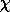that maps elements of the configuration space to n-tuples of real numbers. If there is more than one dimension, the functionis a tuple of n independent coordinate functions19i, i = 0,..., n - 1, where eachi is a real-valued function defined on some region of the configuration space.20 For a given configuration m in the configuration space M the valuesi(m) of the coordinate functions are the generalized coordinates of the configuration. These generalized coordinates permit us to identify points of the n-dimensional configuration space with n-tuples of real numbers.21 For any given configuration space, there are a great variety of ways to choose generalized coordinates. Even for a single point moving without constraints, we can choose rectangular coordinates, polar coordinates, or any other coordinate system that strikes our fancy.

The motion of the system can be described by a configuration path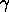mapping time to configuration-space points. Corresponding to the configuration path is a coordinate path q =omapping time to tuples of generalized coordinates. If there is more than one degree of freedom the coordinate path is a structured object: q is a tuple of component coordinate path functions qi =i o. At each instant of time t, the values q(t) = ( q0(t), ..., qn-1(t) ) are the generalized coordinates of a configuration.

The derivative Dq of the coordinate path q is a function22 that gives the rate of change of the configuration coordinates at a given time: Dq(t) = ( Dq0(t), ..., Dqn-1(t) ). The rate of change of a generalized coordinate is called a generalized velocity.

We can make coordinate representations for higher derivatives of the path as well. We introduce the function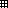(pronounced ``chart'') that extends a coordinate representation to the local tuple:23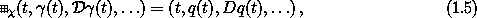where q =o. The functiontakes the coordinate-free local tuple ( t,(t),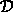(t), ... ) and gives a coordinate representation as a tuple of the time, the value of the coordinate path function at that time, and the values of as many derivatives of the coordinate path function as are needed.

Given a coordinate path q =o, the rest of the local tuple can be computed from it. We introduce a function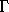that does this: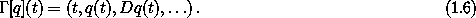The function[q] depends only on the coordinate path q and its derivatives; the function[q] does not depend onor the fact that q is made by composingwith. From relations (1.5) and (1.6) we find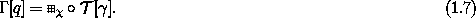Exercise 1.3.  Generalized coordinates
For each of the systems in exercise 1.2, specify a system of generalized coordinates that can be used to describe the behavior of the system.

#### Lagrangians in generalized coordinates

The action is a property of a configuration path segment for a particular Lagrangian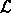. The action does not depend on the coordinate system that is used to label the configurations. We can use this property to find a coordinate representation Lfor the Lagrangian.

The action is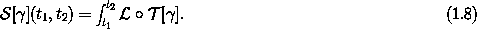The Lagrangianis a function of the local tuple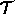[](t) = ( t,(t),(t), ... ). The local tuple has the coordinate representation[q] =o[] , where q =o. So if we choose24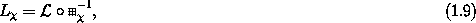then25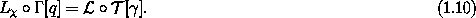On the left we have the composition of functions that use the intermediary of a coordinate representation; on the right we have the composition of two functions that do not involve coordinates. We define the coordinate representation of the action to be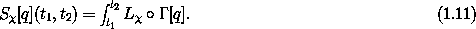The function Stakes a coordinate path; the function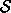takes a configuration path. Since the integrands are the same by equation (1.10), the integrals have the same value: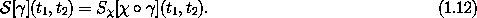So we have a way of constructing coordinate representations of a Lagrangian that gives the same action for a path in any coordinate system.

For Lagrangians that depend only on positions and velocities the action can also be written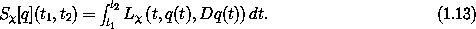The coordinate system used in the definition of a Lagrangian or an action is usually unambiguous, so the subscriptwill usually be dropped.

19 A tuple of functions that all have the same domain is itself a function on that domain: Given a point in the domain, the value of the tuple of functions is a tuple of the values of the component functions at that point.

20 The use of superscripts to index the coordinate components is traditional, even though there is potential confusion, say, with exponents. We use zero-based indexing.

21 More precisely, the generalized coordinates identify open subsets of the configuration space with open subsets of Rn. It may require more than one set of generalized coordinates to cover the entire configuration space. For example, if the configuration space is a two-dimensional sphere, we could have one set of coordinates that maps (a little more than) the northern hemisphere to a disk, and another set that maps (a little more than) the southern hemisphere to a disk, with a strip near the equator common to both coordinate systems. A space that can be locally parameterized by smooth coordinate functions is called a differentiable manifold. The theory of differentiable manifolds can be used to formulate a coordinate-free treatment of variational mechanics. An introduction to mechanics from this perspective can be found in  or .

22 The derivative of a function f is a function, denoted Df. Our notational convention is that D is a high-precedence operator. Thus D operates on the adjacent function before any other application occurs: Df(x) is the same as (Df)(x).

23 The formal definition ofis unimportant to the discussion, but if you really want to know here is one way to do it: First, we define the derivativeof a configuration pathin terms of ordinary derivatives by specifying how it acts on sufficiently smooth real-valued functions f of configurations: (n)(t)(f) = Dn (f o)(t) . Then we define(a, b, c, d, ...) = ( a,(b), c(), d(), ... ). With this definition: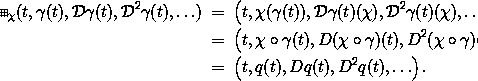24 The coordinate functionis locally invertible, and so is.

25o[] =o-1 oo[] = Lo[o] = Lo[q] .